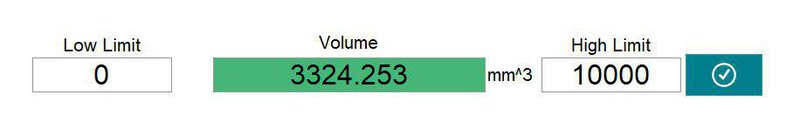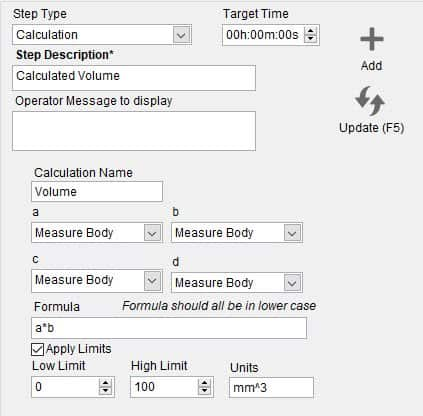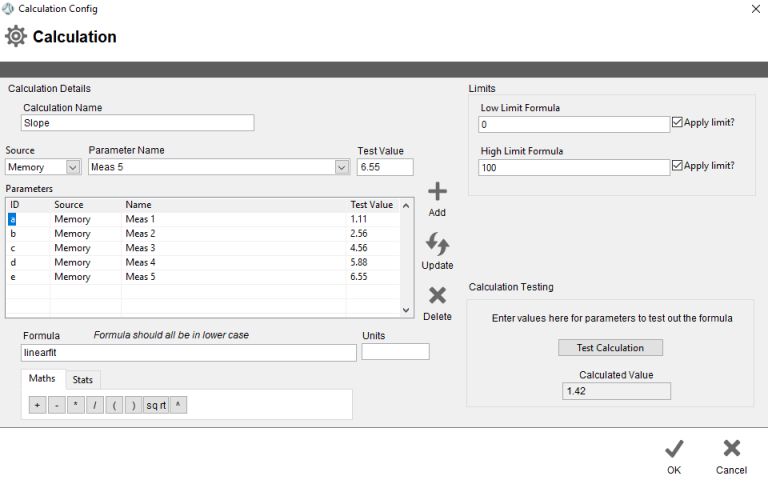# Calculation

This step allows you to perform a mathematical calculation using values entered into Tascus in previous steps.To configure this in the sequence editor, you must select which measurements to use and enter a formula.Use the drop-down lists to select inputs a,b,c & d for the calculation, then enter a formula. The above example shows a formula to multiply inputs a & b: a*b.

To validate your formula, and to edit more complex formulas, click on the Edit button to open the Calculation Editor.The calculation editor allows you to build formulas on the right-hand side, then test a calculation by entering values for a,b,c & d, on the right-hand side.

Function

Details

Maths

+

-

Subtract

*

Multiply

/

Divide

(

Open Brackets

)

Close Brackets

sq rt

Square Root

^

Power of

Stats

Ave

Perfoms a Mean averaging on the dataset

Min

Calculates the Minimum of the dataset

Max

Calculates the Maximum of the dataset

Sum

Calculates the Sum of all the values in the dataset

Sum All

Same function as Sum

Linear Slope

Performs a linear interpolation of the dataset and calculates it's slope